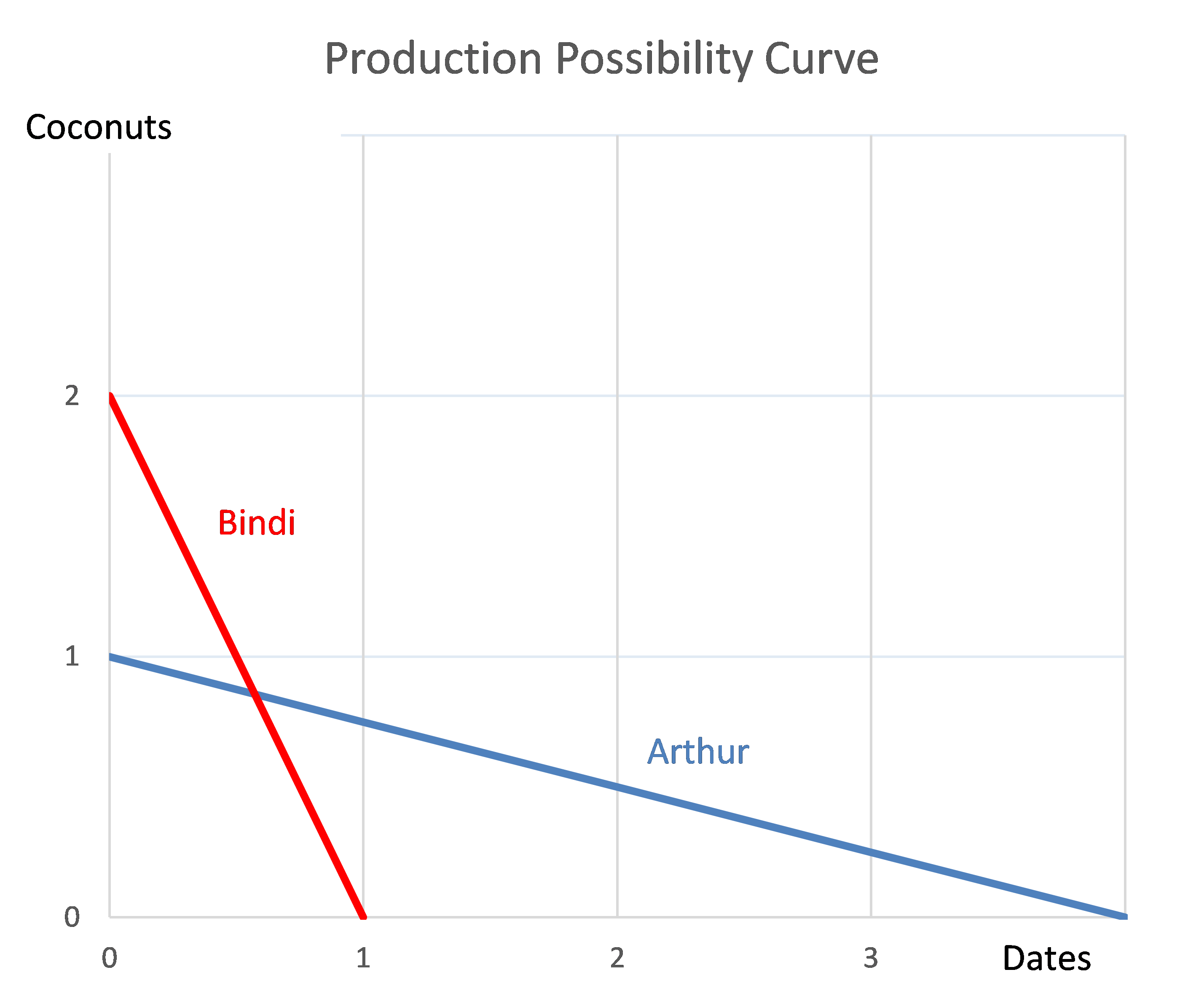# Fight Finance

#### CoursesTagsRandomAllRecentScores

According to the theory of the Capital Asset Pricing Model (CAPM), total variance can be broken into two components, systematic variance and idiosyncratic variance. Which of the following events would be considered the most diversifiable according to the theory of the CAPM?

You have just sold an 'in the money' 6 month European put option on the mining company BHP at an exercise price of $40 for a premium of$3.

Which of the following statements best describes your situation?

Bonds X and Y are issued by different companies, but they both pay a semi-annual coupon of 10% pa and they have the same face value ($100), maturity (3 years) and yield (10%) as each other. Which of the following statements is true? A 10 year bond has a face value of$100, a yield of 6% pa and a fixed coupon rate of 8% pa, paid semi-annually. What is its price?

Will the price of an out-of-the-money put option on equity or if the standard deviation of returns (risk) of the underlying shares becomes higher?

An investor wants to make a portfolio of two stocks A and B with a target expected portfolio return of 16% pa.

• Stock A has an expected return of 8% pa.
• Stock B has an expected return of 12% pa.

What portfolio weights should the investor have in stocks A and B respectively?

What is the correlation of a variable X with itself?

The corr(X, X) or $\rho_{X,X}$ equals:

Fred owns some BHP shares. He has calculated BHP’s monthly returns for each month in the past 30 years using this formula:

$$r_\text{t monthly}=\ln⁡ \left( \dfrac{P_t}{P_{t-1}} \right)$$

He then took the arithmetic average and found it to be 0.8% per month using this formula:

$$\bar{r}_\text{monthly}= \dfrac{ \displaystyle\sum\limits_{t=1}^T{\left( r_\text{t monthly} \right)} }{T} =0.008=0.8\% \text{ per month}$$

He also found the standard deviation of these monthly returns which was 15% per month:

$$\sigma_\text{monthly} = \dfrac{ \displaystyle\sum\limits_{t=1}^T{\left( \left( r_\text{t monthly} - \bar{r}_\text{monthly} \right)^2 \right)} }{T} =0.15=15\%\text{ per month}$$

Assume that the past historical average return is the true population average of future expected returns and the stock's returns calculated above $(r_\text{t monthly})$ are normally distributed. Which of the below statements about Fred’s BHP shares is NOT correct?

The arithmetic average and standard deviation of returns on the ASX200 accumulation index over the 24 years from 31 Dec 1992 to 31 Dec 2016 were calculated as follows:

$$\bar{r}_\text{yearly} = \dfrac{ \displaystyle\sum\limits_{t=1992}^{24}{\left( \ln⁡ \left( \dfrac{P_{t+1}}{P_t} \right) \right)} }{T} = \text{AALGDR} =0.0949=9.49\% \text{ pa}$$

$$\sigma_\text{yearly} = \dfrac{ \displaystyle\sum\limits_{t=1992}^{24}{\left( \left( \ln⁡ \left( \dfrac{P_{t+1}}{P_t} \right) - \bar{r}_\text{yearly} \right)^2 \right)} }{T} = \text{SDLGDR} = 0.1692=16.92\text{ pp pa}$$

Assume that the log gross discrete returns are normally distributed and that the above estimates are true population statistics, not sample statistics, so there is no standard error in the sample mean or standard deviation estimates. Also assume that the standardised normal Z-statistic corresponding to a one-tail probability of 2.5% is exactly -1.96.

Which of the following statements is NOT correct? If you invested \$1m today in the ASX200, then over the next 4 years:

Arthur and Bindi are the only people on a remote island. Their production possibility curves are shown in the graph.Which of the following statements is NOT correct?